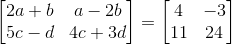Find the values of a, b, c and d from the equations:

Asked by Aaryan | 1 year ago |  201

##### Solution :-

GivenWe know that if two matrices are equal then the elements of each matrices are also equal.

Given that two matrices are equal.

Therefore by equating them we get,

2a + b = 4 …… (1)

And a – 2b = – 3 …… (2)

And 5c – d = 11 …… (3)

4c + 3d = 24 …… (4)

Multiplying equation (1) by 2 and adding to equation (2)

4a + 2b + a – 2b = 8 – 3

⇒ 5a = 5

⇒ a = 1

Now, substituting the value of a in equation (1)

2 × 1 + b = 4

⇒ 2 + b = 4

⇒ b = 4 – 2

⇒ b = 2

Multiplying equation (3) by 3 and adding to equation (4)

15c – 3d + 4c + 3d = 33 + 24

⇒ 19c = 57

⇒ c = 3

Now, substituting the value of c in equation (4)

4 × 3 + 3d = 24

⇒ 12 + 3d = 24

⇒ 3d = 24 – 12

⇒ 3d = 12

⇒ d = 4

a = 1, b = 2, c = 3 and d = 4

Answered by Aaryan | 1 year ago

### Related Questions

#### Find x, y, a and b if

Find x, y, a and b if

#### Find x, y, a and b if

Find x, y, a and b if

#### Construct a 4 × 3 matrix A = [ai j] whose elements ai j are given by ai j = i

Construct a 4 × 3 matrix A = [ai j] whose elements ai j are given by ai j = i

Construct a 4 × 3 matrix A = [ai j] whose elements ai j are given by $$a_{i j} = \dfrac{(i – j)}{(i + j)}$$
Construct a 4 × 3 matrix A = [ai j] whose elements ai j are given by ai j = 2i + $$\dfrac{i}{j}$$